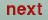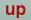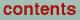Next: Token ALPHA= of cast Up: Category STR Previous: Token KW**2= of cast

### Token IALPHA= of cast integer (optional)

IALPHA=0: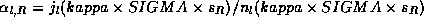is used. Where SIGMA is a token.

IALPHA=1:'s are calculated in the program.and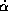depend on kappa. The canonical's only applies for kappa=0. Optimal's (leading to screening for non-zero kappa's) have been calculated as a function of kappa and a fit formula has been found. This is used to calculate the's.

IALPHA=2:(token ALPHA) and(token ADOT) must be supplied in the CTRL file.

O. Jepsen
Thu Oct 12 14:48:45 MESZ 2000